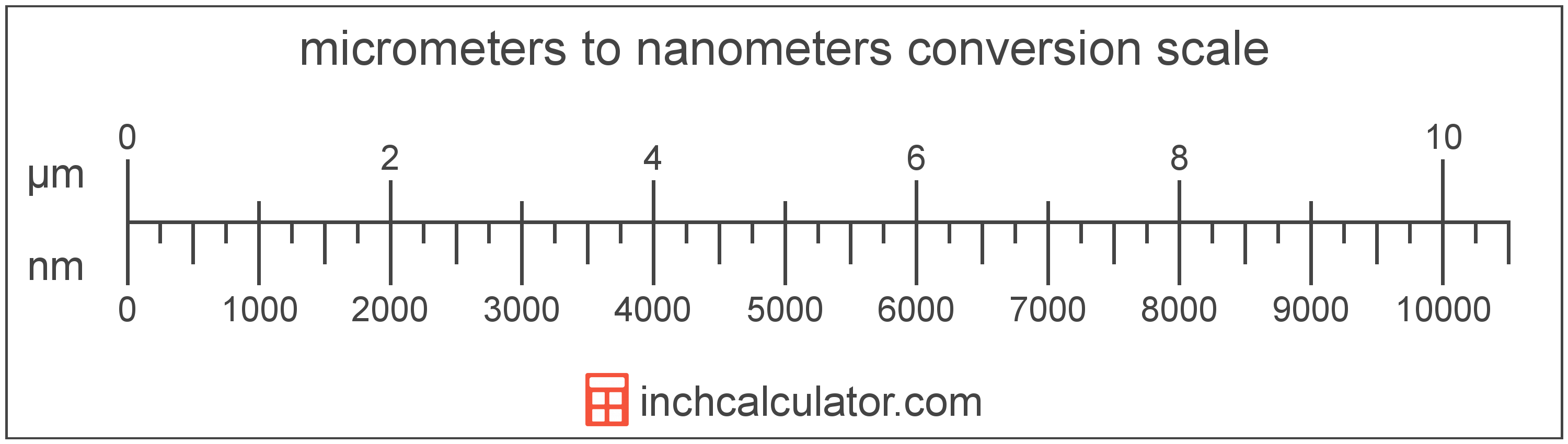# Micrometers to Nanometers Converter

Enter the length in micrometers below to get the value converted to nanometers.

Results in Nanometers:1 µm = 1,000 nm

Do you want to convert nanometers to micrometers?

## How to Convert Micrometers to Nanometers

To convert a measurement in micrometers to a measurement in nanometers, multiply the length by the following conversion ratio: 1,000 nanometers/micrometer.

Since one micrometer is equal to 1,000 nanometers, you can use this simple formula to convert:

nanometers = micrometers × 1,000

The length in nanometers is equal to the length in micrometers multiplied by 1,000.

For example, here's how to convert 5 micrometers to nanometers using the formula above.
nanometers = (5 µm × 1,000) = 5,000 nm### How Many Nanometers Are in a Micrometer?

There are 1,000 nanometers in a micrometer, which is why we use this value in the formula above.

1 µm = 1,000 nm

## What is a Micrometer?

One micrometer is equal to one-millionth (1/1,000,000) of a meter, which is defined as the distance light travels in a vacuum in a 1/299,792,458 second time interval.

The micrometer, or micrometre, is a multiple of the meter, which is the SI base unit for length. In the metric system, "micro" is the prefix for millionths, or 10-6. A micrometer is sometimes also referred to as a micron. Micrometers can be abbreviated as µm; for example, 1 micrometer can be written as 1 µm.

To get an idea of the actual physical length of a micrometer, one human hair is 40-50 µm thick, demonstrating how small this unit of measure is.

## What is a Nanometer?

One nanometer is equal to one-billionth (1/1,000,000,000) of a meter, which is defined as the distance light travels in a vacuum in a 1/299,792,458 second time interval.

The nanometer, or nanometre, is a multiple of the meter, which is the SI base unit for length. In the metric system, "nano" is the prefix for billionths, or 10-9. Nanometers can be abbreviated as nm; for example, 1 nanometer can be written as 1 nm.

The nanometer is an extremely small unit of length measurement, often used to measure things that are very small, such as the transistors and electrical pathways in computer processors and nanotechnology.

We recommend using a ruler or tape measure for measuring length, which can be found at a local retailer or home center. Rulers are available in imperial, metric, or a combination of both values, so make sure you get the correct type for your needs.

Need a ruler? Try our free downloadable and printable rulers, which include both imperial and metric measurements.

## Micrometer to Nanometer Conversion Table

Table showing various micrometer measurements converted to nanometers.
Micrometers Nanometers
0.001 µm 1 nm
0.002 µm 2 nm
0.003 µm 3 nm
0.004 µm 4 nm
0.005 µm 5 nm
0.006 µm 6 nm
0.007 µm 7 nm
0.008 µm 8 nm
0.009 µm 9 nm
0.01 µm 10 nm
0.02 µm 20 nm
0.03 µm 30 nm
0.04 µm 40 nm
0.05 µm 50 nm
0.06 µm 60 nm
0.07 µm 70 nm
0.08 µm 80 nm
0.09 µm 90 nm
0.1 µm 100 nm
0.2 µm 200 nm
0.3 µm 300 nm
0.4 µm 400 nm
0.5 µm 500 nm
0.6 µm 600 nm
0.7 µm 700 nm
0.8 µm 800 nm
0.9 µm 900 nm
1 µm 1,000 nm